1.5.3 The construction application

Presentation

This application is controlled by the application menu on top of the screen (figure 1.24) and the selection menu on bottom of the screen (figure 1.18). It is used to build and manipulate 2D objects made up of points, segments, arcs and splines. Circles and straight lines are only used as construction aids.

• The application uses elementary geometry to define points, straight lines, circles, segments and arcs. For example, by using the tangency property, it is possible to define a circle tangent to three straight lines or a straight line tangent to two circles.
• The application uses splines---a series of points---to define open or closed curves.
• The application is able to cut one object with another object, except the circles and straight lines, which are only construction aids. It is for example possible to cut an object by a straight line. However, the straight line will not be affected by this operation.
• The application is able to round off angles.
• The application is able to duplicate an object n times by using the classical affine transformations: symmetry, rotation, homothety.
• The application is able to "outline" figures.
• The application is able to invert arcs and segments.
• etc.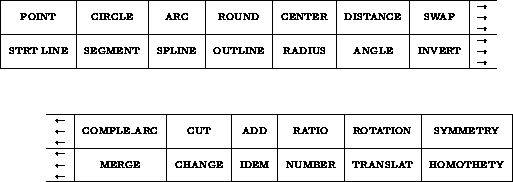Figure 1.24: The CONSTRUCTION application menu

Utilization

Let us recall that points, straight lines, circles, arcs, segments, splines, coordinates (current points, endpoints, etc.) can be selected via the selection menu. We will thus denote by <POINT>  , <LINE>  , <CIRCLE>  , <SEGMENT>  , <ARC>  , <SPLINE>  , <COORD>  the operation of selecting one of these elements or part of it. Furthermore, a <VALUE>  is created by using the calculator or its shortcuts, see sections 1.5.2,1.5.2 for more detail.

Meta-syntax of description of the operations

In the meta-syntax of description of the construction language, we will note xxx  a series of operations defined by <xxx> = ... . ;

We will use the following symbols:

• A B to denote the concatenation of A and B (and) " we wait for A then B".
• A|B to denote an alternative (or) " we wait for A or B"   (this operation has less priority than concatenation).
• * to repeat the ensuing expression 0 or several times.
• " to denote nothing, which in all cases is used to achieve something.
• parentheses ( ) to change priorities.

Example: The expression: <xxx> = ( A | ") *(B|C) E ; can be used to enter character strings beginning or not by A, composed of a series of B or C and ending with E.

Examples of valid strings:

ABBCCCCE               E      AE      CBCBCBCBCBCBCBE
ABBBBBBBE              BE     ABE     BCBCBCBCBCBCBCE
BBBBBBBBBE             CE     ACE     CCCCCCCCCCCCCCE

We will call

• point_coor the selection of POINT or COORD;
• constraint the selection of POINT, COORD, LINE, CIRCLE,
 SEGMENT or ARC;
• elements the selection of POINT, COORD, LINE, CIRCLE,
 SEGMENT, ARC or SPLINE.
• etc.

In the meta-syntax, this reads:

<point_coor> = <POINT>      | <COORD>;
<constraint> = <point_coor> | <LINE> | <CIRCLE> | <ARC>
| <SEGMENT>;
<element>    = <constraint> | <SPLINE>;
In the definition of constraint, the ARC is identified with its supporting circle. Similarly, the SEGMENT is identified with its supporting straight line.

Remark: In the database the elements are described by:

 POINT (a,b) with: x=a; y=b LINE (a,b,c) such that ax+by+c=0 CIRCLE center(a,b) and radius r SEGMENT (P1,P2) with P1=(x1,y1) and P2=(x2,y2) ARC center at P1 passing through P2 and with angle, with P1=(x1,y1) and P2=(x2,y2) SPLINE (number of points, head of the list of points)

At the level of the CONSTRUCTION application, all menus other than the CONSTRUCTION menu are filtered through the applications SELECTION, CALCULATOR, GENERAL and GRAPHIC SCREEN MANAGEMENT. Thus clicking in one of these menus has no direct effect on the application, except possibly changing the selection mode or entering a value. Only the boxes of the application menu and the selections govern the CONSTRUCTION application. For this reason, we will denote by XXXX the operation of clicking in the XXXX box of the current application menu. There will be no confusion with boxes of the same name in other menus.

Example:

• the operation of selecting a circle is denoted CIRCLE
• the operation of clicking in the CIRCLE item of the CONSTRUCTION menu is denoted CIRCLE
Those two "circles" must not be confused with each other. Similarly, if we click in the CENTER item of the selection menu, we will select the center of an arc or a circle which produces a COOR. On the other hand, if we click in the CENTER item of the CONSTRUCTION menu (top), we obtain CENTER.

Modification of the state of the system

The state of the system  is a set of variables used by the application. The global variables of the application are:

• %DISTANCE  used to define a signed distance  which is useful for constructing points, lines, segment, etc.
• %RADIUS  used to define the radius  of an arc or circle, etc.
• %ANGLE  used to define the angle  of an arc or between 2 lines, etc.
• %RATIO  used to define the ratio  of an homothety, etc.
• %NUMBER  used to define the number  of duplications during transformations.
These variables can be modified at almost any moment.

We denote by <state modif>  a modification of the state of the system, which is to say a modification of the value assigned to one of the variable.

<state modif>  = *( <distance> | <radius> | <angle> | <ratio> | <number> )
• Modification of %DISTANCE: <distance>  = DISTANCE ( <VALUE>  | IDEM)
• Modification of %RADIUS: <radius>  = RADIUS (VALUE | IDEM)
• Modification of %ANGLE: <angle>  = ANGLE (VALUE | IDEM)
• Modification of %RATIO: <ratio>  = RATIO (VALUE | IDEM)
• Modification of %NUMBER: <number>  = NUMBER (VALUE | IDEM)

IDEM saves the previous value of the state variable.

Construction of points

POINT
*(  <COORD>
| <POINT>  (  <CIRCLE> | <LINE>
| <ARC>    | <SEGMENT> | <SPLINE>  )
| <LINE>     <element>
| <CIRCLE>   <element>
| <SPLINE>   <element>
);

• First alternative, COORD: Creation of the point of current coordinates by operating the selection menus: MOUSE P, XY POINT, XY FILE, INTERS, EXTREM, MIDDLE, CENTER.

• Other alternatives: We construct a point after having selected two elements.
• If one of the elements is a POINT let point P be the projection of this POINT on the other element
• if this other element is a line we create a point at distance %DISTANCE from P on the line in the direction of the line
• otherwise we create point P.
• If none of the two elements is a POINT, we create the intersection point of the two elements. Since there are in general two points in the intersection of a line and a circle, or of two circle, we choose ``our" point by retaining the one closest to the points used to select the elements.

Examples of point construction

We start by clicking in the POINT box of the construction menu to switch to point construction mode. Then

• we click in the MOUSE P  box of the selection menu. After that, each click in the graphic window constructs a point.
• we click in the XY POINT  box of the selection menu. Each time we enter a couple of numbers on the calculator--- typed on the keyboard and followed by = or (CR) ---we construct a point.
• we click in the XY FILE  box and we enter a file name. A point is constructed for each couple of numbers in the formatted file.
• we construct points of intersection between two elements (line, segment, circle, arc, spline) by selecting a couple of such elements.

Construction of straight lines

LINE  *(  <constraint> <constraint>
| IDEM *<SEGMENT>
);

If the constraints are (the order is irrelevant):

• CircleOrPoint := circle | point_coor;
• CircleOrPoint CircleOrPoint: the line created is tangent to the two circle at the points closest to the ones used to select the two circle
• line line:
• if the two lines intersect, the line created is the bisecting line of the two others.
• if the two lines are equal, we create a line that is parallel to them and at distance %DISTANCE on the side of the selection point.
• otherwise we create a line parallel to the two line at the same distance from both.
• line circle: the line created is parallel to the line and tangent to the circle at a point closest to the one used to select the circle.
• line point_coor: the line created makes an angle %ANGLE with the line and passes through the point_coor.
In the other alternative (IDEM), we construct the line that supports the *segment.

Construction of circles

CIRCLE
*(  <radius> *( CENTER <point_coor> | <constraint> <constraint>)
| <constraint> (  <radius>  <constraint>
| <constraint> ( <radius> | <constraint> ))
| CENTER <point_coor> *(<radius> | <constraint>)
| IDEM *<ARC>
);
Explanation of the various cases (the order is irrelevant):
• radius CENTER point_coor: circle of center at point point_coor and radius

• radius constraint constraint: circle of radius %RADIUS and constrained to be tangent to the two selected elements.

• constraint constraint constraint: circle constrained to be tangent to the three selected elements.

• CENTER point_coor constraint: circle with a given center and tangent to the selected constraint.

• IDEM * arc: circle supported by the * arc (same center and same radius as the *arc).

Construction of arcs

ARC *(  CENTER <point_coor> *(   <angle> <constraint>
| <constraint> (<angle> | <constraint>))
| <radius> *(  CENTER <point_coor>
| <constraint> <constraint> )
| <constraint> (   <radius> <constraint>
| <constraint> (<radius> | <constraint>))
| IDEM *<CIRCLE>
);
Explanation of the various cases (the order is irrelevant). To construct an ARC, we first create a circle satisfying the same constraints and then we create an arc on this circle.
• CENTER  point_coor angle constraint: arc with a given center starting at the point where it is tangent to the constraint and with an angle angle from this point.

• CENTER point_coor radius: arc with a given center, given radius %RADIUS and angle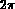• CENTER point_coor constraint constraint: arc with a given center starting at the point where it is tangent to the first constraint, ending at the level of the point where it is tangent to the second constraint and turning counterclockwise.

• radius constraint constraint: arc with a given radius %RADIUS starting at the point where it is tangent to the first constraint, ending at the point where it is tangent to the second constraint.

• constraint constraint constraint: arc tangent to the three
 constraint and linking the three points of tangency in the order of the constraint.

• IDEM *circle: creates an arc of angle 2on each selected circle.

Construction of segments

SEGMENT *( <constraint> <constraint> );
Explanation of the various cases (the order is irrelevant).

To construct a SEGMENT, we first create the LINE that has the same constraints. Then we construct a segment on this line connecting the two points of tangency to the two constraints if they exist. Otherwise, we use the state variable %DISTANCE.

Thus for example:

• circle circle: The segment is tangent to the two circle (at the points closest to the points used to select the two circle).

• line line:
• if the lines intersect, the segment is the bisecting line of the two line and is of length %DISTANCE.
• if the two lines are equal, we create a segment that is parallel to them and at distance %DISTANCE on the side of the selection point. The segment is of length %DISTANCE or of the same length as line depending on whether line is a LINE or a SEGMENT.
• otherwise we create a segment parallel to the two line at the same distance from both. It is of length %DISTANCE.

• line circle: The segment is parallel to the selected line and is tangent to the circle at the point closest to the one used to select the circle. It is of length %DISTANCE and starts at the point of tangency with the circle.

Construction of splines

SPLINE <point> *<point>;
Constructs the cubic spline  passing through the points. We can create a closed spline by making the last point equal to the first point (by selecting it).

Geometrical transformations

ROTATION , TRANSLAT , HOMOTHETY , SYMMETRY  all define transformations that can be applied on selected elements.

ROTATION    <state modif> ( CENTER | " ) <point_coor> <state modif> *<element>
TRANSLAT    <point_coor> <point_coor>    *<element>
HOMOTHETY  <state modif> CENTER (<point_coor> | " ) <state modif>   *<element>
SYMMETRY   (<point_coor> | <LINE>| <SEGMENT>)   *<element>
ROTATION
applies the rotation of center point and angle %ANGLE to the
elements. If %NUMBER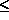1, the elements are moved, otherwise %NUMBER-1 copies of each element are created. Therefore, after transformation there are %NUMBER elements on the screen.

TRANSLAT
applies to the elements the translation by a vector defined by the first point and the second point. If %NUMBER1, the elements are moved, otherwise %NUMBER-1 copies of each element are created. Therefore, after transformation there are %NUMBER elements on the screen.

HOMOTHETY
applies the homothety of center point and ratio %RATIO to the elements. If %NUMBER1, the elements are moved, otherwise %NUMBER-1 copies of each element are created. Therefore, after transformation there are %NUMBER elements on the screen.

SYMMETRY
applies the symmetry with respect to point or line on all selected elements. Warning: if %NUMBER is larger than 2, elements will be superimposed on one another.

Rounding off angles

This command is used to round off angles  between SEGMENTS and ARCS.

ROUND  *( <constraint> <constraint> )
We round off an angle between the two constraint with radius %RADIUS, the rounded angle will always turn counterclockwise. A rounded angle is an arc whose supporting circle is tangent to the two constraint. The arc starts at the first point of tangency and ends at the second point of tangency, turning counterclockwise. If one of the constraint is an ARC or a SEGMENT, this constraint is modified in such a way that the element terminates at the point of tangency with the rounded angle.

Defining a contour

CONTOUR   (<LINE> | <CIRCLE>)  (<LINE> | <CIRCLE>)
(<LINE> | <CIRCLE>) (COMPLE_ARC | ")
*( (<LINE> | <CIRCLE>) (COMPLE_ARC | ")      )
This command is used to define a contour  formed by SEGMENTS and ARCS supported by a set of straight lines and circles. The program functions by calculating the intersection of constraint i with constraint i+1, which gives point I, then the intersection of constraint i+1 with constraint i+2, which gives point I+1. I and I+1 form a new portion of the contour and so on.

Let us give an example with straight lines (figure 1.25):

Let us assume that we have five lines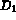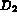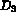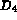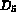that intersect at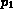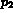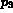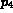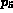, and that we wish to define the contour composed of the segments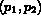,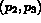,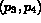,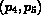and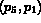. We denote by +i the ith selected point.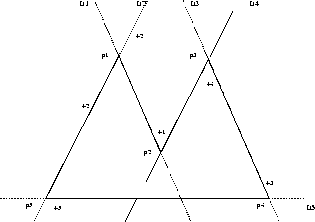Figure 1.25: Example of contour definition

We selectat +2 thenat +1 which definesThen we selectat +4 which defines(at this point, the program drawsThen we selectat +3 which defines(at this point, the program drawsThen we selectat +5 which defines(at this point, the program drawsThen we selectat +2 which defines(at this point, the program drawsThen we selectat +1 which defines(at this point, the program drawsIn the case of circles, we must be careful because the selection points are used to remove ambiguities of multiple intersections: the selection point of the i constraint is used to remove the selection ambiguity between constraints i and i+1.

Reversing segments or arcs

REVERSE *( <ARC> | <SEGMENT> )

Reverses  a SEGMENT or an ARC

• ARC :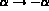• SEGMENT: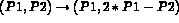(Symmetry / P1)

Complementing arcs

COMPLE_ARC *ARC
Changes an ARC into its complement  if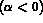then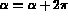otherwise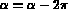Inverting elements

INVERT *(<ARC> | <SEGMENT> | <SPLINE>)

Inverts  an ARC, SEGMENT or a SPLINE

• ARC :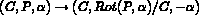• SEGMENT: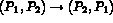• SPLINE :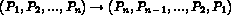Cutting one element with another?

CUT  * (  <point_coor> *(<ARC> | <SEGMENT> | <SPLINE>)
| (<LINE> | <CIRCLE> | <ARC> | <SEGMENT> | <SPLINE>)
(<LINE> | <CIRCLE> | <ARC> | <SEGMENT> | <SPLINE>)
)
If we first select point, we cut  an ARC, a SEGMENT or a SPLINE in two by the projection of point on the element.
For the SPLINE, we cut at the definition point closest to point point.
Otherwise we cut the selected elements with one another if they are divisible (non divisible elements are LINE and CIRCLE).

Changing part of an element

CHANGE *(  ANGLE <ARC> (<VALUE> | IDEM)
| RADIUS <CIRCLE> (<VALUE> | IDEM)
| <POINT> <point_coor>)
This command is used to change  :
• the angle of a selected ARC
• the radius of a CIRCLE
• the position of a point (the first point is changed into the second one. The first point can be the center of an arc or a circle)
• the extremity of a SEGMENT or an ARC.
• a definition point of a SPLINE, thus the shape of the SPLINE.

ADD *(  POINT   *( <ARC> | <SEGMENT> | <SPLINE> | <POINT> <POINT> )
| SEGMENT *( <SEGMENT> <SEGMENT> )
| SPLINE  *( <SPLINE> (   <SPLINE> | *<POINT>  ) )
| ARC     *( <ARC> <ARC>  )

• If POINT, we add  %NUMBER points with a ratio %RATIO on ARC or SEGMENT or SPLINE or between the two POINTS.
• If SEGMENT, we add %NUMBER segments defined by linear interpolation of ratio %RATIO between the two SEGMENTS.
• If SPLINE
• followed by the selection of two SPLINES, then we add %NUMBER splines defined by linear interpolation of ratio %RATIO between the two SPLINES.
• followed by the selection of one SPLINE and one POINT, then we add new definition POINTS given by *point to the selected SPLINE.

• If ARC, we add %NUMBER arcs defined by 3 points (2 extremities and the middle point) defined by linear interpolation of ratio %RATIO between the extremities and the middle points of the 2 selected arcs.

Merging two elements

MERGE *( <segment> <segment> | <arc> <arc> | <spline> <spline> )

This command is used to merge  2 SEGMENTS, 2 ARCS or 2 SPLINES that have a common extremity into a single element of the same type. The resulting element has the same orientation as the first selected element. This instruction is only executed if the following conditions are met:

• in the case of 2 segments, both segments must be colinear.
• in the case of 2 arcs, both arcs must have the same center and the same radius.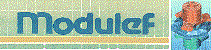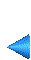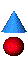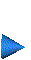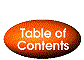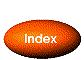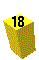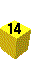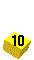Next: The PREP_MESH application Up: The EMC˛ preprocessor Prev: 1.5.2 The global menus Index Contents